Courses

# Thermodynamics & Power Plant - 1

## 15 Questions MCQ Test GATE Mechanical (ME) 2022 Mock Test Series | Thermodynamics & Power Plant - 1

Description
This mock test of Thermodynamics & Power Plant - 1 for GATE helps you for every GATE entrance exam. This contains 15 Multiple Choice Questions for GATE Thermodynamics & Power Plant - 1 (mcq) to study with solutions a complete question bank. The solved questions answers in this Thermodynamics & Power Plant - 1 quiz give you a good mix of easy questions and tough questions. GATE students definitely take this Thermodynamics & Power Plant - 1 exercise for a better result in the exam. You can find other Thermodynamics & Power Plant - 1 extra questions, long questions & short questions for GATE on EduRev as well by searching above.
QUESTION: 1

### Gas from a cylinder of compressed Argon is used to inflate an inelastic balloon, originally folded completely to a volume of 1 m3. If the barometer reads 760 mm of Hg. What is the amount of work done upon the atmosphere by the balloon?

Solution: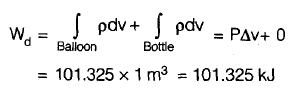QUESTION: 2

### An ideal gas undergoes an isothermal expansion from state R to state S in a turbine as shown in the diagram given below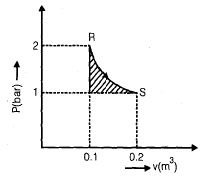The area of shaded region is 1000 N-m, what is the amount of turbine work done during the process?

Solution: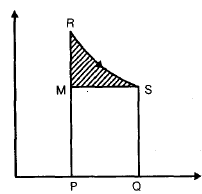Turbine work = area of PQSR

= area of RMS + area of MSPQ

= 1000 + (2 - 1) x 105 (0.2 - 0.1)

= 1000 + 10,000

= 11000 N-m

QUESTION: 3

### The work done by a closed system in a reversible process is always ___ that done in an irreversible process.

Solution:
QUESTION: 4

A stationary mass of gas is compressed without friction from an initial state of 0.3 m3 and 0.105 MPa to a final state of 0.15 m3 and 0.105 MPa the pressure remains constant throughout the process. There is a transfer of 37.6 kJ of heat from the gas during the process. How much does the internal energy of the gas changes?

Solution: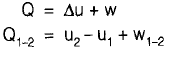= 0.105 (0.15 - 0.30)MJ

w1_2 = -15.75 kJ

Q1-2 = 37.6 kJ

- 37.6 = Δu - 15.75

u2 - u1 = -21.85 kJ

QUESTION: 5

A refrigerator having COP 5 is used for heating purpose in winter so that atmosphere becomes the cold body and room to be heated becomes hot body, how much heat would be available for heating for each kW input to the driving motor. Electromechanical efficiency of motor is 90%.

Solution:

W= 0.9 x 1 kW
= 0.9 kW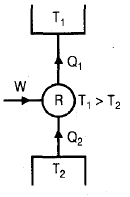COP =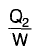Q2 = 5 X 0.9

= 4.5 kW

Q1= Q2 + W = 4.5 + 0.9

= 5.4 kW

*Answer can only contain numeric values
QUESTION: 6

Two reversible engines operate between thermal reservoirs at 1200 K, T 2 K and 300 K such that first engine receives heat from 1200 K reservoir and rejects heat to thermal reservoir at T2K, while the second engine at 300 K. The efficiency of both the engines is equal then, the value of temperature T2 is _________ k.

Solution:

When the efficiency of both the engines are equal then intermediate temperature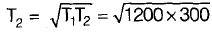= 600 K

QUESTION: 7

Saturated liquid at a higher pressure P1 having h11 = 1000 kJ/kg is throttled to a lower pressure P2 . The enthalpy of saturated liquid and saturated vapour are 800 kJ/kg and 2800 kJ/kg respectively. Find the dryness fraction of vapour after throttling.

Solution:

In throttling process enthalpy remains constant.

h1 = h2

1000 = 800 + x(2800 — 800)

x = 0.1.

QUESTION: 8

The system is partitioned in two equal parts. An intensive property of each part will

Solution:

An intensive property is not depended on mass of the system.

QUESTION: 9

The molar heat capacity of a certain substance varies with temperature according to the empirical equation C = (27.2 + 4 × 10–3 T) J/mol-K How much heat is necessary to change the temperature of 2 mole of this substance from 27°C to 427°C.

Solution:

T i = 300 k

T f = 700 k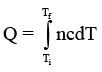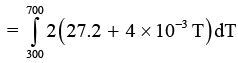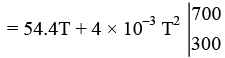= 23360 J = 23.3 kJ

QUESTION: 10

For a real thermodynamic cycle, which one of the following is correct?

Solution:

From the Clausius inequality for real thermodynamic cycle,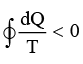QUESTION: 11

When a system is in equilibrium, any conceived change in entropy would be

Solution:
*Answer can only contain numeric values
QUESTION: 12

The loss of available energy associated with the transfer of 1000 kJ of heat from a constant temperature system of 600 k to another at 400 k when the atmospheric temperature is 300 k, is ________ kJ.

Solution:

Loss of available energy =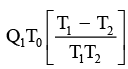= 1000 × 300 =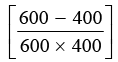= 250 kJ

QUESTION: 13

One kilogram of water at room temperature is brought into contact with a high temperature thermal reservoir. The entropy change of the universe is

Solution:

For every irreversible process entropy of the universe increases.

QUESTION: 14

A thermal power plant operates on a regenerative cycle with a single open feed water heater, as shown in figure. For the state points shown, the specific enthalpies are: h1 = 2800 kJ/kg and h2 = 200 kJ/kg. The bleed to the feed water heater is 20% of the boiler steam generation rate. The specific enthalpy at state 3 is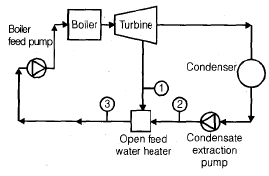Solution:

Enthalpy at state 3 = h3

= 0.2 h 1 + 0.8 h2

= (0.2 × 2800) + (0.8 × 200)

= 506 + 160 = 720 kJ/kg

QUESTION: 15

Two vapour power cycles having efficiencies 0.50 and 0.40 are coupled in series then the efficiency of coupled cycle will be

Solution:

We know that for two cycle coupled in series η = 1 – (1 + η1 ) (1 – η2 )
= 1 – [1 – η1 – η2 + η1 η2 )
= η1 + η2 – η1 η2
= 0.5 + 0.4 – 0.5 × 0.4 = 0.70